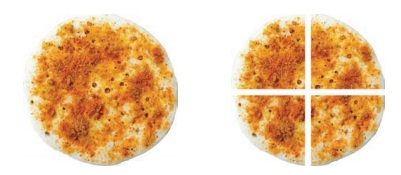Home | | Maths 5th Std | Types of fractions

# Types of fractions

Proper fraction: In a fraction, if the numerator is smaller than the denominator, then it is called as a proper fraction. Examples: 3/5, 4/15, 9/21. Improper fraction: In a fraction, if the numerator is greater than the denominator, then it is called as an improper fraction. Examples: 9/5, 11/3, 21/17

Types of fractions:

Represent the following figure in fraction.

It is 3 /8. In this, 3 is the numerator which is smaller than the denominator, that is, 3 < 8.

This type of fraction is called as proper fraction.Proper fraction: In a fraction, if the numerator is smaller than the denominator, then it is called as a proper fraction

Examples: 3/5, 4/15, 9/21.

Improper fraction: In a fraction, if the numerator is greater than the denominator, then it is called as an improper fraction.

Examples: 9/5, 11/3, 21/17

Mixed fraction:

Kavitha had five dosas. She wanted to share the dosas among herself and her 3 friends equally. Each one got a dosa. She didn’t know how to divide the remaining one dosa equally.

So, she requested her teacher to help. The teacher asked her to divide the remaining 1 dosa into 4 parts, so that each one would get 1 out of 4 parts. Teacher explained her how much did each one of them get out of 5 dosas. That is, each one got 1 and 1 /4 dosas.Also, she told her that these type of fractions are called mixed fractions.

A fraction which contains a whole number and a proper fraction is called as a mixed fraction. In the above situation, 1 is the whole number and 1/4 is the proper fraction.

Tags : Fractions | Term 3 Chapter 6 | 5th Maths , 5th Maths : Term 3 Unit 6 : Fractions
Study Material, Lecturing Notes, Assignment, Reference, Wiki description explanation, brief detail
5th Maths : Term 3 Unit 6 : Fractions : Types of fractions | Fractions | Term 3 Chapter 6 | 5th Maths